# Heat Transfer by Natural Convection (Part - 3) Chemical Engineering Notes | EduRev

## Chemical Engineering : Heat Transfer by Natural Convection (Part - 3) Chemical Engineering Notes | EduRev

The document Heat Transfer by Natural Convection (Part - 3) Chemical Engineering Notes | EduRev is a part of the Chemical Engineering Course Heat Transfer for Engg..
All you need of Chemical Engineering at this link: Chemical Engineering

Illustration 5.1

A hot oven is maintained at 180 oC having vertical door 50 cm high is exposed to the atmospheric air at 20oC. Calculate the average heat transfer coefficient at the surface of the door.

The various air properties at the average temperature [(180+20)/2 = 100oC] are,

k = 0.032 W/m oC;      Pr = 0.7;          Kinematic viscosity = 24 x 10-6 m2/s

At Tb = 20oC,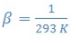Solution 5.1

First we have to find the Grashof number,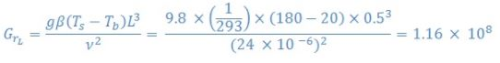With the help of Gr and Pr, we can estimate the Ra number,

Ra = GrPr = 1.16 X 108 X 0.7 = 8.12 X 107

As Ra < 109, the eq.5.7 can be used,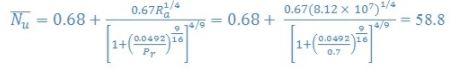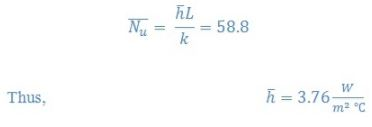5.3 Combined natural and forced convection

As we know that there is hardly any situation in which only natural or forced convective heat transfer occurs. Generally, in all the processes natural and forced convection heat transfer occur but depending upon the contribution made, the process may be approximated as either natural or forced convection problem.  However, certain situation needs to be addressed as combined natural and forced convection problem. The following is a thumb rule to determine the individual situations.

1. Forced convection region i.e. negligible natural convection contribution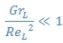2. Natural convection region i.e. negligible forced convection contribution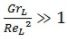3. Mixed convection i.e., significant contribution by both natural and forced convections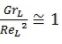In this situation, the following equation may be used,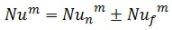where, Nu is the Nusselt number due to mixed convection, Nun is the Nusselt number due to natural convection, and Nuf is the Nusselt number due to forced convection. The value of m is generally taken as 3, whereas positive and negative signs can be used for the convection in the same and opposite directions, respectively.

Illustration 5.2

In the oven door described in illustration 5.1 is subjected to an upward flow of air (that is forced convection). What would be the minimum free stream velocity for which natural convection may be neglected?

Solution 5.2.

Section 5.3 above shows that for the following condition the effect of natural convection may be neglected,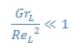The value of Gr number calculated in the previous illustration was 1.16 X 108

Thus,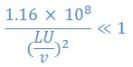U >> 0.24 m/s

Therefore, the bulk velocity of the air should be far greater that 0.24 m/s.

Offer running on EduRev: Apply code STAYHOME200 to get INR 200 off on our premium plan EduRev Infinity!

## Heat Transfer for Engg.

46 videos|60 docs|59 tests

,

,

,

,

,

,

,

,

,

,

,

,

,

,

,

,

,

,

,

,

,

;